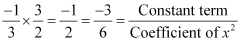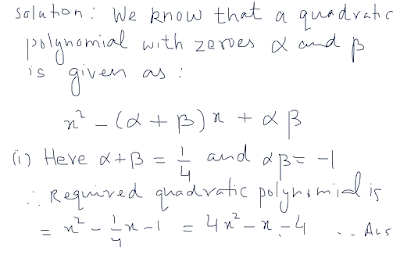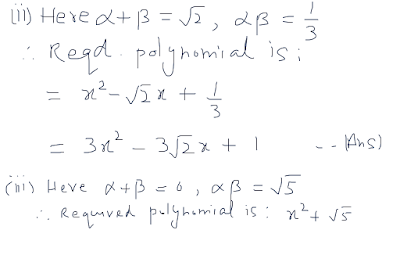## PolynomialsNCERT Solutions Ex 2.2

Q1: Find the zeroes of the following quadratic polynomials and verify the relationship between the zeroes and the coefficients.
(i)  x2-2x-8
(ii) 4s2-4s+1
(iii) 6x2-3-7x
(iv) 4u2+8u
(v) t2-15
(vi) 3x2 - x -4

Answer: (i)  x2-2x-8 = (x - 4)(x +2)
⇒x2-2x-8 = 0 when x - 4= 0 or x + 2 = 0
∴ Zeroes of x2- 2x - 8 are 4 and -2.
Sum of zeroes =Product of zeroes =Hence relationship between zeroes and coefficients are verified.

(ii) 4s2-4s+1
= (2s -1 )2
⇒ 4s2-4s+1 = 0 when 2s -1 = 0
⇒ s = 1/2
∴ Zeroes of 4s2-4s+1 are ½ and ½.
Sum of zeroes =Product of zeroes =Hence relationship between zeroes and coefficients are verified.

(iii) 6x2-3-7x
= (3x + 1)(2x - 3)
⇒ 6x2-3-7x = 0 when 3x + 1 = 0 OR 2x - 3 = 0
⇒ x = -1/3 or x = 3/2 ∴ Zeroes of 6x2-3-7x are -1/3 and 3/2
Sum of zeroes =Product of zeroes =Hence relationship between zeroes and coefficients are verified.

(iv) 4u2+8u
= 4u(u + 2)
⇒ 4u2+8u = 0 when 4u = 0 OR u + 2 = 0
⇒ u = 0 OR u = -2
∴ Zeroes of 4u2+8u are 0 OR -2
Sum of zeroes =Product of zeroes =Hence relationship between zeroes and coefficients are verified.

(v) t2-15
= t2 - 0t -15 = (t - √15)(t + √15)
⇒ The value of t2-15 is 0 when t - √15 = 0 OR t + √15 = 0
⇒ t = √15 or t = -√15.
Sum of zeroes = √15 -√15 = 0
Product of zeores = (√15) ☓ (-√15) = -15
Hence relationship between zeroes and coefficients are verified.

(vi) 3x2 - x -4
= 3x2 - 3x + 4x -4 = 3x(x + 1) - 4(x + 1) = (x + 1)(3x - 4)
⇒ The value of 3x2 - x -4 is 0, when x + 1 = 0 OR 3x -4 = 0
⇒ x = -1 OR x = 4/3
Sum of zeroes = -1 + 4/3 = -1/3
Product of zeroes = -1 ☓ 4/3 = -4/3
Hence relationship between zeroes and coefficients are verified.

Q2: Find a quadratic polynomial each with the given numbers as the sum and product of its zeroes respectively.
(i) 1/4, -1
(ii) √2, 1/3
(iii) 0, √5
(iv) 1,1
(v)-1/4, 1/4
(vi) 4,1